Date: Fri, 5 Dec 1997 22:58:01 -0600 (CST)
Subject: calculating intersection point

Name: Milton

Question:

Please help me. I work for a gov. agency and need help for my work. Given 2 lines through (x1,y1),(x2,y2),and (x3,y3),(x4,y4). Can you give me a good formula for calculating the intersection point. I would greatly appreciate it.Thanks.

Hi Milton

There are a couple of ways of presenting a procedure for finding the point of intersection of two lines. The easiest way to proceed is in two steps, write the equations for the two lines and then solve them to find the point P of intersection. In your case the lines are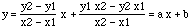and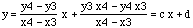.

Let these two equations define a, b, c and d then solving the equations gives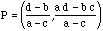.

If you want you can write the coordinates of P in terms of the coordinates of the original points as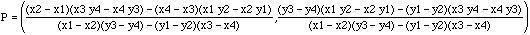.

You can also express P in terms of determinants as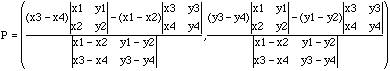.

Cheers,
Chris and Penny

Go to Math Central